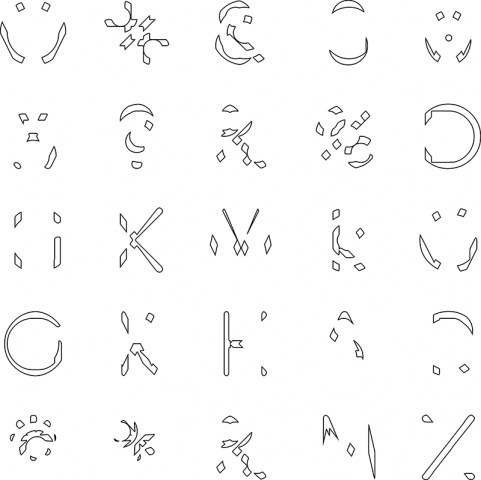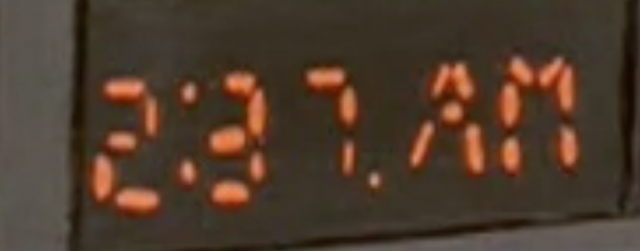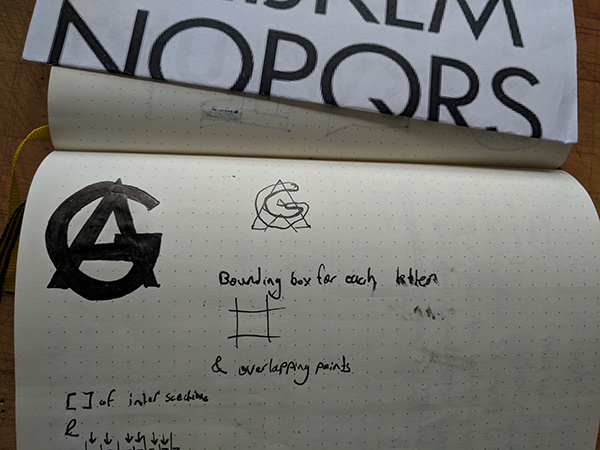# tyvan-Asemic

///A Page from Another Planet//

Proportions define the style and readability of a typeface, so it is important to understand the relationships among characters for type designers. This project’s input is a set of characters, chosen or random, and outputs the overlapping areas of those characters. When writing the code, I initially generated random characters for each overlap, but
many of the characters had minimal overlap, which did not lead to interesting results. Here, the characters were chosen based on size, form, and overlap percentage. This program can be easily adapted, with an additional function, to output a grid of  proportions of the characters. This project studies intersections to understand approaching the creation of a segmented typeface.
More of my studies for segmented type design: http://bit.do/SegType// Unedited plotter vids:```import geomerative.*; import processing.pdf.*;   // 9x12 plottor dimensions   RFont font; String frstCHAR = "A"; String scndCHAR = "G"; String typeFace = "eurof55.ttf"; int typeSize = 135;     // [str][str] Letter One and Letter Two char[][]chosenLetters = new char;     //char[][]chosenLetters = //{{'W', 'Q'}, {'#', '%'}, {'@', '&amp;'}, {'8', 'G'}, {'0', 'W'},   // {'W', '5'}, {'@', '?'}, {'%', '&amp;'}, {'@', '%'}, {'Q', 'D'},   // {'G', 'H'}, {'K', 'X'}, {'W', 'M'}, {'@', 'k'}, {'W', 'G'},   // {'G', 'O'}, {'R', 'W'}, {'H', 'K'}, {'A', 'S'}, {'Q', 'R'},   // {'@', '*'}, {'*', '%'}, {'%', '&amp;'}, {'M', 'N'}, {'Z', 'X'}, };     //----------------SETUP--------------------------------- void setup() { size(800, 800); //smooth(8); RG.init(this); font = new RFont(typeFace, typeSize, CENTER); noLoop();     // Uncomment for random letters for (int i = 0; i &lt; chosenLetters.length; i++) { for (int j = 0; j &lt; chosenLetters[i].length; j++) { char text = chooseLetters(); chosenLetters[i][j] = text; } } }   //----------------DRAW--------------------------------- void draw() {   // exports PDF // beginRecord(PDF, "line.pdf"); //boolean charOneFill = true; //boolean charTwoFill = true; background(255); translate(-100, 0);   // Organize the grid of characters for (int i = 0; i &lt; chosenLetters.length; i++) { int xNum = 5; int lineLeading = typeSize; int tracking = typeSize; if (i == 0) { pushMatrix(); } else if (i%xNum == 0) { popMatrix(); translate(0, lineLeading); pushMatrix(); } else { translate(tracking, 0); }   // Should this function be called something else? // golanDoit[first letter][ second letter] golanDoit(chosenLetters[i], chosenLetters[i]); }   // PDF end of page //endRecord(); }     // Randomly choose which characters intersect char chooseLetters() { int lOne = int(random(48, 90)); char lONE = (char)(lOne); return lONE; }       // Adapted by Golan Levin and Ty Van de Zande // from the tutorial by Ricard Marxer // http://www.ricardmarxer.com/geomerative/ // www.freeartbureau.org/blog //http://www.gnu.org/licenses/gpl.html     void golanDoit (char c1, char c2) { pushMatrix(); translate(200, 200);   RCommand.setSegmentLength(2); RCommand.setSegmentator(RCommand.UNIFORMLENGTH);   RShape aCharacterOne = font.toShape(c1); RPoint[] characterPointsOne = aCharacterOne.getPoints();   RShape aCharacterTwo = font.toShape(c2); RPoint[] characterPointsTwo = aCharacterTwo.getPoints();   RShape outputShape = RG.intersection(aCharacterOne, aCharacterTwo); RPoint[] outputPoints = outputShape.getPoints();   if (outputPoints != null) { int nOutputPoints = outputPoints.length; println("nOutputPoints = " + nOutputPoints);     strokeWeight(1);   //fill(0); stroke(0, 0, 255, 100); //beginShape(); //for (int i=0; i&lt;characterPointsOne.length; i++) { // vertex(characterPointsOne[i].x, characterPointsOne[i].y); //} //endShape();   //stroke(255, 0, 0, 100); //beginShape(); //for (int i=0; i&lt;characterPointsTwo.length; i++) { // vertex(characterPointsTwo[i].x, characterPointsTwo[i].y); //} //endShape();   stroke(1); strokeWeight(1); float prevX = 0; float prevY = 0; for (int i=0; i&lt;nOutputPoints; i++) { float currX = outputPoints[i].x; float currY = outputPoints[i].y; float d = dist(currX, currY, prevX, prevY); if (d &lt; 4.0) { line(currX, currY, prevX, prevY); }   prevX = currX; prevY = currY; } }   popMatrix(); }```